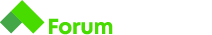# Problem 4. Loops C#

Здравейтем имам проблем със следната задача :

# Min Max Sum Average

## Description

Write a program that reads from the console a sequence of N real numbers and returns the minimal , the maximal number, the sum and the average of all numbers (displayed with 2 digits after the decimal point).

• The input starts by the number N (alone in a line) followed by N lines, each holding an real number.
• The output is like in the examples below.

## Input

• On the first line, you will receive the number N .
• On each of the next N lines, you will receive a single real number.

## Output

• You output must always consist of exactly 4 lines - the minimal element on the first line, the maximal on the second, the sum on the third and the average on the fourth, in the following format:
``````min=3.00
max=6.00
sum=9.00
avg=4.50
``````

## Constraints

• 1 <= N <= 1000
• All numbers will be valid integer numbers that will be in the range `[-10000, 10000]`

## Sample tests

Input Output
3

2
5
1|min=1.00
max=5.00
sum=8.00
avg=2.67|
|3
2
-1
4|min=-1.00
max=4.00
sum=5.00
avg=1.67|

Първите няколко теста минава след това, гърми… Ето и кода ми :

В условието на задачата пише, че могат да бъдат реални числа, което означава, че може да не са всички цели. Когато промениш това, би трябвало да проработиКакто е писала Иоана числата са реални.